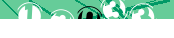# JavaScript: Generating Random Numbers

While the JavaScript Math library includes a method for generating random numbers, it's not always clear how to incorporate it into your code.

## Math.rand() function

Here's a sample of random numbers generated by your browser. You should see a range of floating point numbers with up to 16 decimal places (less in some browsers):

The numbers generated are in a range from 0 to <1 or, in mathematical notation, [0,1).

## Generating a random integer

In this example, we're going to simulate the rolling of a standard six-sided die. In other words, generate an integer between 1 and 6 inclusive.

#### First attempt:

` var rand = Math.round(Math.random() * 6);`

Interestingly, this can also be written as: ``` with(Math) { var rand = round(random() * 6); }```

The round method rounds a floating point number to the nearest integer.

#### Result:

We've generated and plotted 500 random 'rolls' of the die using the above code:

0123456

As you can see, we don't end up with an even six-way spread as you might expect. Instead you see that '0' and '6' receive around half as many results as 1 through 5, so using round isn't the way to go.

#### A better method:

` var rand = Math.floor(Math.random() * 6);`

The floor method rounds numbers down to the nearest integer.

#### Result:

Already the results look much better:

0123456

#### Best practice:

The only thing left to do is to shift the values to the right.

` var rand = 1 + Math.floor(Math.random() * 6);`

#### Result:

Finally, we've achieved our goal:

0123456

The JavaScript documentation describes the random method as a pseudo-random number generator as in some situations the results can be predictable. If you need truly random numbers you are better off using a server-side language such as PHP.

## Dice-rolling function

Now that we're clear on how to generate random integers, how about simulating rolls of multiple dice. This is probably an outdated concept now, but at one time there were thousands of people using dice with different numbers of sides for role-playing and other games.

```<script type="text/javascript"> // Original JavaScript code by Chirp Internet: chirpinternet.eu // Please acknowledge use of this code by including this header. function rollDie(sides) { if(!sides) sides = 6; with(Math) return 1 + floor(random() * sides); } function rollDice(number, sides) { var total = 0; while(number-- > 0) total += rollDie(sides); return total; } </script>```

The functions above can be called as follows:

``` var rand = rollDie(); # d6 var rand = rollDie(6); # d6 var rand = rollDie(20); # d20 var rand = rollDice(3); # 3d6 var rand = rollDice(3, 6); # 3d6 var rand = rollDice(2, 12); # 2d12```

## Working Example

The graph below shows the result of 1,000 rolls of three six-sided dice (3d6):

The result should be a familiar bell curve.

## References

< JavaScript

#### Send a message to The Art of Web:<- copy the digits from the image into this box

press <Esc> or click outside this box to close

13 February, 2020

Steve, the chances of Math.random() being 0 are like guessing a number between 1 and 100 quadrillion correctly, but there's no shame in being safe. I probably would too honestly, but I use the randojs.com library to cover all my bases for me and make things simple. To pick a number from 1 to 3, for example, you'd just have to write:

`rando(1, 3)`

could be worth a look if you're tired of writing out these wordy random lines all the time.

20 December, 2018

Math.random() * 6 will get you a value 0-6 inclusive of 0 but not of 6. Rounding here doesn't make sense, which is why your first graph is "wrong".

16 May, 2015

I just looked at your javascript random number generator. I wanted to generate a number from 1-3 with even odds and used ceil. What I was wondering about was whether I could ever get a 0 -- since random could conceivably generate a 0, this looked conceivable, so I added code to cover that (admittedly rare) case. You didn't worry about it -- am I being overly cautious or are you being too loose?

You should use floor and add 1 to the output:
`var rand = 1 + Math.floor(Math.random() * 3);`

Otherwise you will encouter a rare zero value.

top